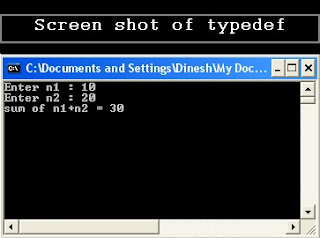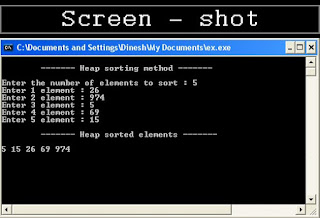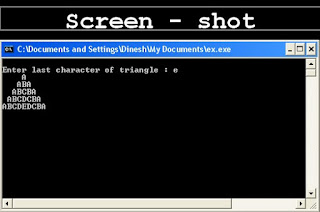## 2.29.2012

### typedef datatype

Q . Write and explain the significance of "typedef datatype" in c programming with example.

Ans.

In C typedef is a keyword.

Keyword typedef stands for type definition.

It is used for create an alias name of existing data type. typedef is used by writing the keyword typedef, followed by the existing data type and then the new name.

Syntax:

typedef existing_daya_type new_name ;

Example:

typedef char ch;

In above example, i has defined ch as char, so now i could perfectly use ch as declarations of any other valid types:

ch yourchar;
ch mychar,*str;

Note:- typedef does not create different types. It only create synonyms of existing data types i.e. in our example the type of mychar can be considered to be either ch or char, since both are in fact the same type.

Example of typedef:

typedef int num;
num n1,n2,n3;

/*c program for shows the use of typedef data type */
#include<stdio.h>
#include<conio.h>
int main()
{
typedef int number;
number n1,n2,sum;
printf("Enter n1 : ");
scanf("%d",&n1);
printf("Enter n2 : ");
scanf("%d",&n2);
sum=n1+n2;
printf("sum of n1+n2 = %d",sum);
getch();
return 0;
}

/*************** OUTPUT ***************/Example of typedef

typedef can be useful to define an alias for a type that is frequently used within a program.

typedef is also useful to define types when it is possible that we will need to change the type in later our program.

typedef is also used when existing_name is too large or confusing.

Related post:

1. enum
2. struct
3. union

## 2.24.2012

### Heap sorting

Q. Write a C program to sort 5 numbers using heap sorting method.

Ans.

/* c program for heap sorting method*/

#include<stdio.h>
#include<conio.h>
void manage(int *int);
void heapsort(int *intint);
int main()
{
int arr;
int i,j,size,tmp,k;
printf("\n\t------- Heap sorting method -------\n\n");
printf("Enter the number of elements to sort : ");
scanf("%d",&size);
for(i=1; i<=size; i++)
{
printf("Enter %d element : ",i);
scanf("%d",&arr[i]);
manage(arr,i);
}
j=size;
for(i=1; i<=j; i++)
{
tmp=arr;
arr=arr[size];
arr[size]=tmp;
size--;
heapsort(arr,1,size);
}
printf("\n\t------- Heap sorted elements -------\n\n");
size=j;
for(i=1; i<=size; i++)
printf("%d ",arr[i]);
getch();
return 0;
}

void manage(int *arr, int i)
{
int tmp;
tmp=arr[i];
while((i>1)&&(arr[i/2]<tmp))
{
arr[i]=arr[i/2];
i=i/2;
}
arr[i]=tmp;
}

void heapsort(int *arr, int i, int size)
{
int tmp,j;
tmp=arr[i];
j=i*2;
while(j<=size)
{
if((j<size)&&(arr[j]<arr[j+1]))
j++;
if(arr[j]<arr[j/2])
break;
arr[j/2]=arr[j];
j=j*2;
}
arr[j/2]=tmp;
}

/************* OUTPUT ***************/Screen shot for Heap sorting C program

Related programs:

## 2.21.2012

### Number rhombus2

Q. Write a C program to print the number in following fashion:
1
212
32123
4321234
543212345
4321234
32123
212
1

Ans.

/*c program for number pyramid*/
#include<stdio.h>
#include<conio.h>
int main()
{
int num,r,c,sp;
printf("Enter number of rows : ");
scanf("%d",&num);
for(r=1; r<=num; r++)
{
for(sp=num-r; sp>0; sp--)
printf(" ");
for(c=r; c>=1; c--)
printf("%d",c);
for(c=2; c<=r; c++)
printf("%d",c);
printf("\n");
}
for(r=1; r<=num; r++)
{
for(sp=r; sp>=1; sp--)
printf(" ");
for(c=num-r; c>=1; c--)
printf("%d",c);
for(c=2; c<=num-r; c++)
printf("%d",c);
printf("\n");
}
getch();
return 0;
}

/*************** OUTPUT ***************/

## 2.20.2012

### Merge sorting

Q. Write a C program to that sort 5 numbers using merge sorting.

Ans.
/* c program for merge sorting */

#include<stdio.h>
#include<conio.h>
void merge(int [],int ,int ,int );
void part(int [],int ,int );
int main()
{
int arr;
int i,size;
printf("\n\t------- Merge sorting method -------\n\n");
printf("Enter total no. of elements : ");
scanf("%d",&size);
for(i=0; i<size; i++)
{
printf("Enter %d element : ",i+1);
scanf("%d",&arr[i]);
}
part(arr,0,size-1);
printf("\n\t------- Merge sorted elements -------\n\n");
for(i=0; i<size; i++)
printf("%d ",arr[i]);
getch();
return 0;
}

void part(int arr[],int min,int max)
{
int mid;
if(min<max)
{
mid=(min+max)/2;
part(arr,min,mid);
part(arr,mid+1,max);
merge(arr,min,mid,max);
}
}

void merge(int arr[],int min,int mid,int max)
{
int tmp;
int i,j,k,m;
j=min;
m=mid+1;
for(i=min; j<=mid && m<=max ; i++)
{
if(arr[j]<=arr[m])
{
tmp[i]=arr[j];
j++;
}
else
{
tmp[i]=arr[m];
m++;
}
}
if(j>mid)
{
for(k=m; k<=max; k++)
{
tmp[i]=arr[k];
i++;
}
}
else
{
for(k=j; k<=mid; k++)
{
tmp[i]=arr[k];
i++;
}
}
for(k=min; k<=max; k++)
arr[k]=tmp[k];
}

/************* OUTPUT *****************/Screen shot for merge sort C program

Related programs:

## 2.19.2012

### Symbol rhombus

Q. Write a C program to print the following number structure:
*
***
*****
*******
*****
***
*

Ans.

/*c program for symbol pyramid*/

#include<stdio.h>
#include<conio.h>
int main()
{
int num,r,c,sp;
printf("Enter number of rows : ");
scanf("%d",&num);
for(r=1; r<=num; r++)
{
for(sp=num-r; sp>=1; sp--)
printf(" ");
for(c=1; c<=r; c++)
printf("*");
for(c=r-1; c>=1; c--)
printf("*");
printf("\n");
}
for(r=1; r<=num; r++)
{
for(sp=r; sp>=1; sp--)
printf(" ");
for(c=1; c<=(num-r); c++)
printf("*");
for(c=num-r-1; c>=1; c--)
printf("*");
printf("\n");
}
getch();
return 0;
}

/*********** OUTPUT ************/

### Number rhombus

Q.  Write a C program to print following number rhombus.

1
121
12321
1234321
123454321
1234321
12321
121
1

Ans.

/*c program for number rhombus structure*/

#include<stdio.h>
#include<conio.h>
int main()
{
int num,r,c,sp;
printf("Enter number of rows : ");
scanf("%d",&num);
for(r=1; r<=num; r++)
{
for(sp=num-r; sp>=1; sp--)
printf(" ");
for(c=1; c<=r; c++)
printf("%d",c);
for(c=r-1; c>=1; c--)
printf("%d",c);
printf("\n");
}
for(r=1; r<=num; r++)
{
for(sp=r; sp>=1; sp--)
printf(" ");
for(c=1; c<=(num-r); c++)
printf("%d",c);
for(c=num-r-1; c>=1; c--)
printf("%d",c);
printf("\n");
}
getch();
return 0;
}

/**************************************************
The output of above program would be:
**************************************************/Figure: Screen shot of Number rhombus C program

You might also like to read:

## 2.17.2012

### Character rhombus

Q. Write a C program to print character in following fashion.
OR
Write a C program to print a character rhombus.
A
ABA
ABCBA
ABCDCBA
ABCBA
ABA
A

Ans.

/*c program to print character rhombus structure*/
#include<stdio.h>
#include<conio.h>
int main()
{
char ch,r,c,chh;
int sp;
printf("\nEnter any character : ");
scanf("%c",&ch);
if(ch>='a' && ch<='z')
ch=ch-32;
printf("\n");
for(r='A'; r<=ch; r++)
{
for(sp=ch-r; sp>=1; sp--)
printf(" ");
for(c='A'; c<=r; c++)
printf("%c",c);
for(c=r-1; c>='A'; c--)
printf("%c",c);
printf("\n");
}
for(r='A'; 'A'<=ch; ch--,r++)
{
for(sp=r; sp>='A'; sp--)
printf(" ");
for(c='A'; c<=ch-1; c++)
printf("%c",c);
for(c=ch-2; c>='A'; c--)
printf("%c",c);
printf("\n");
}
getch();
return 0;
}

/********** OUTPUT ***********/CHARACTER RHOMBUS

## 2.15.2012

### Square triangle4

Q. write a C program to display the character in following fashion:
A
BA
CBA
DCBA
EDCBA
DCBA
CBA
BA
A

Ans.

/* c program for square character triangle */

#include<stdio.h>
#include<conio.h>
int main()
{
char ch,r,c;
int sp;
printf("\nEnter last character of triangle : ");
scanf("%c",&ch);
if(ch>='a' && ch<='z')
ch=ch-32;
printf("\n");
for(r='A'; r<=ch; r++)
{
for(c=r; c>='A'; c--)
printf("%c",c);
printf("\n");
}
for(r='A'; 'A'<=ch; ch--,r++)
{
for(c=ch-1; c>='A'; c--)
printf("%c",c);
printf("\n");
}
getch();
return 0;
}

/************* OUTPUT **************/

### Square triangle3

Q. write a C program to display the character in following fashion:
A
AB
ABC
ABCD
ABCDE
ABCD
ABC
AB
A
Ans.

#include<stdio.h>
#include<conio.h>
int main()
{
char ch,r,c;
int sp;
printf("\nEnter last character of triangle : ");
scanf("%c",&ch);
if(ch>='a' && ch<='z')
ch=ch-32;
printf("\n");
for(r='A'; r<=ch; r++)
{
for(sp=ch-r; sp>=1; sp--)
printf(" ");
for(c='A'; c<=r; c++)
printf("%c",c);
printf("\n");
}
for(r='A'; 'A'<=ch-1; ch--,r++)
{
for(sp=r; sp>='A'; sp--)
printf(" ");
for(c='A'; c<ch; c++)
printf("%c",c);
printf("\n");
}
getch();
return 0;
}

/***************** OUTPUT ********************/

### Square triangle2

Q. write a C program to display the character in following fashion:

ABCDCBA
ABCBA
ABA
A
Ans.

#include<stdio.h>
#include<conio.h>
int main()
{
char ch,r,c;
int sp;
printf("\nEnter last character of triangle : ");
scanf("%c",&ch);
if(ch>='a' && ch<='z')
ch=ch-32;
printf("\n");
for(r='A'; 'A'<=ch; ch--,r++)
{
for(sp=r; sp>'A'; sp--)
printf(" ");
for(c='A'; c<=ch; c++)
printf("%c",c);
for(c=ch-1; c>='A'; c--)
printf("%c",c);
printf("\n");
}
getch();
return 0;
}

/*************** OUTPUT**************/

### Square triangle1

Q. write a C program to display the character in following fashion:

A
ABA
ABCBA
ABCDCBA

Ans.

#include<stdio.h>
#include<conio.h>
int main()
{
char ch,r,c;
int sp;
printf("Enter last character of triangle : ");
scanf("%c",&ch);
if(ch>='a' && ch<='z')
ch=ch-32;
for(r='A'; r<=ch; r++)
{
for(sp=ch-r; sp>=1; sp--)
printf(" ");
for(c='A'; c<=r; c++)
printf("%c",c);
for(c=r-1; c>='A'; c--)
printf("%c",c);
printf("\n");
}
getch();
return 0;
}

/***************** OUTPUT ******************/Character triangle